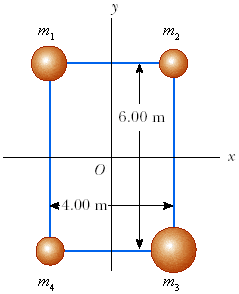# Center Gravity and Moment of Inertia

• ymehuuh
In summary, the conversation is discussing finding the moment of inertia of a system of four objects held in a rectangle by light rods. The mass values of the objects are given and the task is to find the moment of inertia about the x axis, y axis, and an axis through point O perpendicular to the page. The solution involves finding the center of gravity and using the equation Σmr2 to calculate the moment of inertia. Pythagoras' theorem can also be used to simplify the calculations.

## Homework Statement

Four objects are held in position at the corners of a rectangle by light rods as shown in the figure below. The mass values are given below.
M1 (kg) M2 (kg) M3 (kg) M4 (kg)
3.50 1.50 3.90 1.70(a) Find the moment of inertia of the system about the x axis.

(b) Find the moment of inertia of the system about the y axis.

(c) Find the moment of inertia of the system about an axis through O and perpendicular to the page.

## Homework Equations

Center of Gravity: sumM1*X1+M1*X2.../M1+M2...

## The Attempt at a Solution

I found the center of gravity for the x-axis to be .037736...how do I find the moment of inertia with that information?

#### Attachments

Last edited:
You need to find the x and y CoM.

Knowing that then the moment I about the CoM is Σmr2.

The axes are simpler in that for

I_x = Σm_i*y_i2

I_y = Σm_i*x_i2

Oh, I reversed the two and that's why I got it wrong. Thanks.
What would I use for part c?

ymehuuh said:
Oh, I reversed the two and that's why I got it wrong. Thanks.
What would I use for part c?

What are the distances to each corner from O to each mass?

I_o = Σmr2

It's made a little easier by Pythagoras, so be sure and thank him.

lowlypion said:
what are the distances to each corner from o to each mass?

I_o = Σmr2

it's made a little easier by pythagoras, so be sure and thank him.

perfect! Thanks!